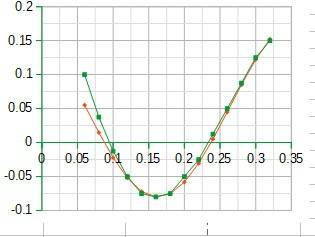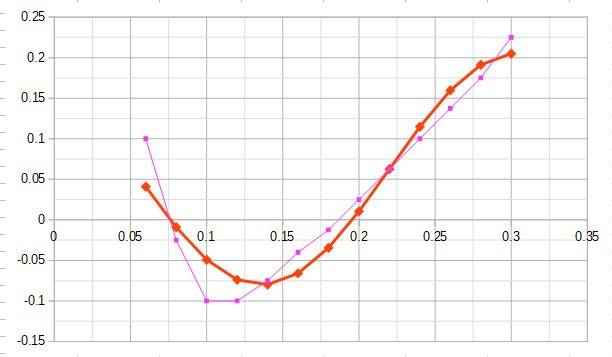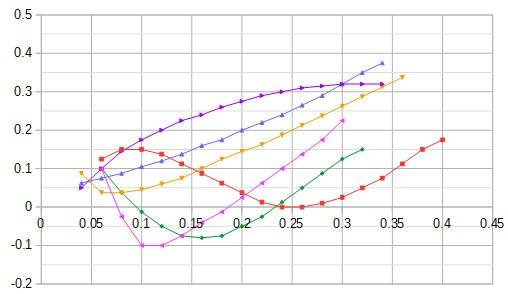# Determining the Equation of a Sine and Cosine Graph that speeds up

• I
FGD
TL;DR Summary
What is the equation I would need to fit my function to the graph?
My function needs to speed up towards the left. How do I do this?Green is the graph.
Red is my function that needs to match the graph.
A = Amplitude = -0.13
H = Phase Shift = 0.1625
V = Vertical Shift = 0.05
P = period = 0.4
B = 2π / P
Y = A (Cos(B (X-H) ) + V

Homework Helper
Gold Member
Using that function, there is no way to "speed up" the left side without changing the right side. The parameter B controls the "speed" evenly across the entire thing.

FGD
Is there another function that would work?

Homework Helper
Gold Member
My immediate answer is to treat the two sides separately, using the same function but changing B. Is that ok? For other ideas, you should specify any additional requirements that you have.

FGD
Good suggestion. You just gave me another thought. Would there be some way to alter B based on X?
The additional requirements are that this data set will be compared against multiple with the same function. My worst fit is this data set. (Again faster frequency on the left. Red is my function)Homework Helper
Gold Member
Good suggestion. You just gave me another thought. Would there be some way to alter B based on X?
The additional requirements are that this data set will be compared against multiple with the same function. My worst fit is this data set. (Again faster frequency on the left. Red is my function)View attachment 264304
I don't know a good way to change B smoothly based on x, because that would change the right side. What I had in mind was something where you leave B with its current value when x > H and give it a larger value when x < H.

FGDHere is the full data set. The order is Red, Green, Pink, Yellow, Blue, Purple. If I can get this function to work then I hopefully can get each data set to morph into the next over time.

Homework Helper
Gold Member
FGD
Yeah something very similar to the bicubic example in that link.